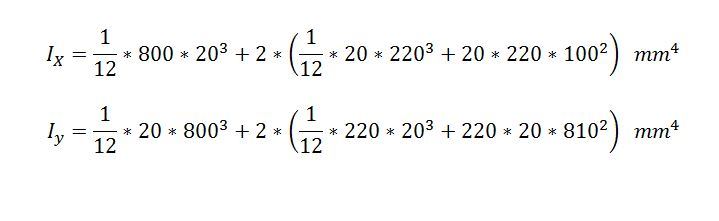The International Information Center for Structural Engineers

Wednesday, 04 October 2017 11:49

## Calculation Example – Calculate shear stress for temperature load.

A copper bar with a rectangular cross section is held without stress between rigid supports. The temperature of the bar is raised 50°C. Determine the maximum shear stress t. (Assume α= 17.5 x 10-6/°C and E =120 GPa.)

Wednesday, 20 September 2017 11:49

## Calculation Example – Calculate the moments of inertia Ix and Iy.

Determine the moments of inertia Ix and Iy of the Z section. The origin of coordinates is at the centroid.

SolutionMonday, 21 August 2017 11:18

## Calculation Example - Calculate the Axial Forces of the Truss Members.

Find the axial forces of the members 2-3, 9-3 of the truss for the given loads.

Thursday, 20 July 2017 12:49

## Calculation Example – Internal forces.

Determine the internal forces at point C.

Monday, 26 June 2017 12:24

## Calculation Example – Determine the magnitudes of F1,F2.

Determine the magnitudes of F1,F2 and the angle Θ so that the external forces and the external moment create a zero resultant force and moment at the wheel (Radius=3m, units KN,m, degrees).

Tuesday, 23 May 2017 09:13

## Calculation Example – Determine the shear force and moment.

Determine the shear force and moment acting at a section passing through point C on the beam.

Wednesday, 03 May 2017 10:44

## Calculation Example - Calculate the deflection. Castigliano Theorem.

Calculate the deflection at point D for the point load 2P for the pinned beam at two ends.

Tuesday, 25 April 2017 00:00

## Calculation Example – Allowable shear force for the girder.

Calculate the maximum allowable shear force Vmax for the girder. The welded steel girder is having the cross section shown in the figure. It is fabricated of two 300 mm x30 mm flange plates and a 300 mm x 30 mm web plate. The plates are joined by four fillet welds that run continuously at the length of the girder. Each weld has an allowable load in shear of 1000 kN/m.

Monday, 03 April 2017 12:18

## Calculation Example - Annular cross section, Stress.

A strain gage is located at D on the surface of the rod AB. The average normal stress on the rod is σ when the rod is subjected to forces F as on the sketch. Assume that the cross section at D is annular and the outer diameter DEXT, calculate the inner diameter DINT of the cross section at D.

Monday, 13 March 2017 00:00

## Calculation Example – Plane stress.

Calculate the stresses acting on an element oriented at an angle θ= 60° from the x axis, where the angle θ is positive when counterclockwise.

Page 3 of 6
• Default
• Title
• Date
• Random
• ## Keller lays foundation for Aston Martin tower

Keller business units HJ Foundation and Hayward Baker worked on the foundation system of one of Miami's most prestigious, eye-catching